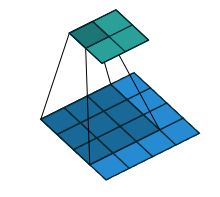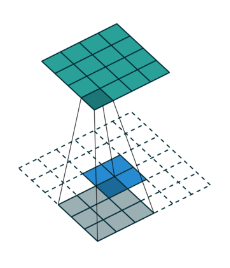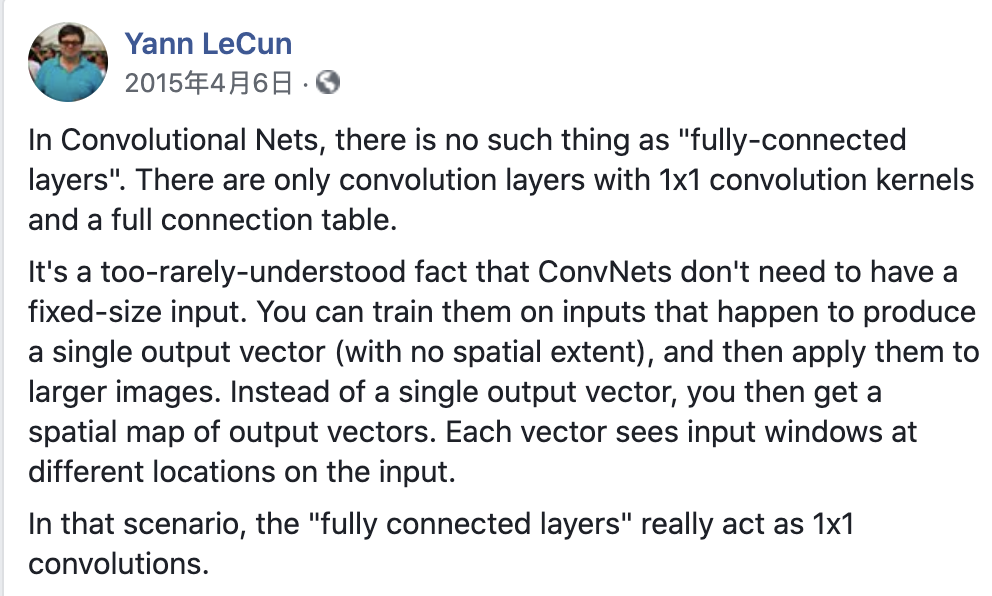## 概述

Long等人提出的全卷积网络实现了端到端、像素到像素的语义分割：全卷积网络（Fully Convolutional Network）之所以全（Full），是因为最基础的FCN设计真的只有卷积和他的衍生操作，这其中就包括著名的转置卷积。

While a general deep net computes a general nonlinear function, a net with only layers of this form computes a nonlinear ﬁlter, which we call a deep ﬁlter or fully convolutional network. An FCN naturally operates on an input of any size, and produces an output of corresponding (possibly resampled) spatial dimensions.

## 转置卷积 Transposed Conv

P.S. 下面利用构造的稀疏矩阵实现了正向卷积，事实上，im2col可以更有效地（相比这种方法）将正向卷积问题转换为通用矩阵相乘问题（GEMM），这也是Caffe实现卷积的方法。

K = np.arange(1, 10).reshape((1,1,3,3))
conv = torch.nn.Conv2d(in_channels=1, out_channels=1, kernel_size=3)
custom_K = torch.Tensor(K)
conv.weight = torch.nn.Parameter(custom_K)
conv.bias = torch.nn.Parameter(torch.Tensor(np.zeros(1)))
print(conv.weight)

X = np.arange(1, 17).reshape((1,1,4,4))
X_T = torch.Tensor(X)

print(conv(X_T))
# 传统Conv采用互相关运算

W, k = np.zeros((4, 16)), np.zeros(11)   #  4 -- 输出的2x2  16 -- 输入flatten之后的长度
k[:3], k[4:7], k[8:] = K[0, 0, 0, :], K[0, 0, 1, :], K[0, 0, 2, :]
W[0, 0:11], W[1, 1:12], W[2, 4:15], W[3, 5:16] = k, k, k, k

# W的构造方法参考托普利兹矩阵

np.dot(W, X.reshape(16)).reshape((1, 1, 2, 2)), W

# 转换为矩阵乘法后与互相关结果相同
# 结果：
# Parameter containing:
# tensor([[[[1., 2., 3.],
#           [4., 5., 6.],
#           [7., 8., 9.]]]], requires_grad=True)
# tensor([[[[348., 393.],
#           [528., 573.]]]], grad_fn=<MkldnnConvolutionBackward>)
# (array([[[[348., 393.],
#           [528., 573.]]]]),
#  array([[1., 2., 3., 0., 4., 5., 6., 0., 7., 8., 9., 0., 0., 0., 0., 0.],
#         [0., 1., 2., 3., 0., 4., 5., 6., 0., 7., 8., 9., 0., 0., 0., 0.],
#         [0., 0., 0., 0., 1., 2., 3., 0., 4., 5., 6., 0., 7., 8., 9., 0.],
#         [0., 0., 0., 0., 0., 1., 2., 3., 0., 4., 5., 6., 0., 7., 8., 9.]]))B(可以理解为滤波器)=[9,4] (Toeplitz matrix)

B的转置为[4,9]

## 思维转换——全连接层看作卷积层

$1 \times 1$ 卷积，顾名思义就是卷积核大小仅为$1 \times 1$ 的卷积层，在实际运用中是很好的降维手段，且与全连接层输出固定长度的向量不同，$1 \times 1$ 卷积层仍有卷积层的性质：输出大小与输入相关。

• 1x1卷积在输入尺寸为1x1时，与全连接层的数学原理没有区别：拿我们入门的MLP分类为例，假设最后一层隐藏层大小Flatten之后为1x1x512，经过(1x1x512)x10的1x1卷积后输出1x1x10，10即为Num of classes。
• 当输入为HxWxC时，全连接层等价于有n个HxWxC卷积核的卷积层。FCN就巧妙利用了这一结构，将分类网络中的全连接层换做$1 \times 1$ 卷积层，结合某些up-sampling方法的特性，可以在最后得到与输入相同大小的heatmap。

## Shift-and-stitch

Shift-and-stitch是从coarse的输出中得到dense prediction的方法之一，这个方法的名字十分直观：

We ﬁnd learning through upsampling, as described in the next section, to be more effective and efﬁcient, especially when combined with the skip layer fusion described later on.

## Patchwise training v.s. Fully convolutional training

Fully convolutional training很好理解，就是将分类网络中的全连接层换成卷积层。但这种方式有一个问题：对图片中每个像素的预测都接受了整个图片作为输出，而实际上就经验而言，预测一个像素的类别并不需要这么多信息。Patchwise Training则是在前馈过程中选择图片周围的小patch，可能起到帮助收敛、平衡类别的效果。

Whole image fully convolutional training is identical to patchwise training where each batch consists of all the receptive ﬁelds of the units below the loss for an image (or collection of images). 一句提及了两者的相关性。

We ﬁnd that sampling does not have a signiﬁcant effect on convergence rate compared to whole image training, but takes signiﬁcantly more time due to the larger number of images that need to be considered per batch.

4.3部分提到，sampling对收敛没啥用，但还额外花了不少时间，所以之后的实验都是Fully Convolutional Training了。

## 总结与感受体会

Last modification：June 7th, 2020 at 04:56 pm
If you think my article is useful to you, please feel free to appreciate